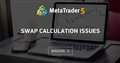# How to Calculate Swap?280

Hi. This post is a continuation, of sorts, to the thread started here: https://www.mql5.com/en/forum/218952 . It was titled "Swap Calculation
issues..." by BoredMember but the thread was hijacked by the "rules", so many of us never got to see a solution. I am approaching the issue in a
different way, so I have made some alterations to the original code and left the original comments intact. In short, this is my attempt to
present a solution. I have used some exaggerated values in the example below to illustrate the steps taken to arrive at the result. If I were to
use actual values then each user will most likely get a different result value.

For clarity, I think it is best to set this solution in an example which would be similar to the likely usage situation. Now, the general
formula to calculate swap is:
Swap = PipValue * Lots * Swaprate * NumberOfNights.

Example 1 - We have a position open with the following details:
Lots=1(100000 EUR).
Order Type= OP_SELL
Duration= 1 rollover night.

I need to know what is the entire swap at rollover for this position, so I constructed the following tools to assist me:

```updated on 4.4.19

//+------------------------------------------------------------------+
//| GetPipValue
//+------------------------------------------------------------------+
/*
Get a symbol's pip value in terms of Account currency.
*/
double GetPipValue(string SymbolPair, double Position_Lots, double AccountCurrency_Exchange_Rate)
{
double pvalue = 0;
double pip = 0.0001;
string BaseCurrency = SymbolInfoString(SymbolPair,SYMBOL_CURRENCY_BASE);
{
pvalue = (pip/askp) * Position_Lots * contractsize;//this is in Base Currency
pvalue = pvalue / AccountCurrency_Exchange_Rate;
}
else
{
Print("Failed to get ASK price for the symbol "+SymbolPair+" for GetPipValue. Please ensure the symbol is listed in MarkeWatch and that you are connected to the trade server.");
}
return pvalue;
}
//+------------------------------------------------------------------+
//| end of GetPipValue
//+------------------------------------------------------------------+

//+------------------------------------------------------------------+
//| GetSwapRateInAccountCurrency
//+------------------------------------------------------------------+
/*
Get a symbols swap rate in account currency.
*/
double GetSwapRateInAccountCurrency(string aSymbol,int aOrderType, double ExchangeRate)
{
// now check swap type
int swapType=MarketInfo(aSymbol, MODE_SWAPTYPE);
double swapRate=0;

// get out the tick value to calculate swap points
double tickValue=MarketInfo (aSymbol, MODE_TICKVALUE);  // tick value in deposit currency (typically USD)
string accntcurrency_str = AccountInfoString(ACCOUNT_CURRENCY);

//Swap calculation method. 0 - in points; 1 - in the symbol base currency; 2 - by interest; 3 - in the margin currency
double AccntCurrency=0;
if (aOrderType==OP_SELL)
{
swapRate=SymbolInfoDouble(aSymbol,SYMBOL_SWAP_SHORT);
}
{
swapRate=SymbolInfoDouble(aSymbol,SYMBOL_SWAP_LONG);
}
if (swapType==0)
{
AccntCurrency = swapRate/ExchangeRate;
}
else if (swapType==1)
{
// need to convert to account currency
// get currency of symbol
string base_currency_Str=SymbolInfoString(aSymbol,SYMBOL_CURRENCY_BASE);
AccntCurrency = swapRate/ExchangeRate;
}
else if (swapType==2)
{
// interest rate or percentage version, e.g. bitcoin. Use %tage of price but returned down to a day. Probably wrong...
AccntCurrency=((swapRate/100)*MarketInfo(aSymbol, MODE_BID))/365;
}
else if (swapType==3)
{
// this is easiest. Amount is in margin currency which = account currency
AccntCurrency=swapRate;
}

return(AccntCurrency);
}
//+------------------------------------------------------------------+
//| end of GetSwapRateInAccountCurrency
//+------------------------------------------------------------------+
```

Inorder to calculate swap we need to get the value for the following variables to plug into the formula above:

PipValue = ?

Lots = ?

Swaprate = ?

NumberOfNights = ?

Let's do this in mql4:

```double PipValue = GetPipValue("EURUSD", 1, 1);
double Lots = 1;
double Swaprate = GetSwapRateInAccountCurrency("EURUSD",OP_SELL,1);
int NumberOfNights = 1;```

The above evaluates to(The values below are not real):

```PipValue = 3;
Lots = 1;
Swaprate = 8;
NumberOfNights = 1;```

Thus, Swap = 3 * 1 * 8 * 1 = 24\$USD.

Maybe someone could correct/change/confirm.Swap Calculation issues
• 2017.11.06
• www.mql5.com
Hi, I know this has come up many times before but I still cannot work out a generic way to calculate the account currency swap (typically USD) for...